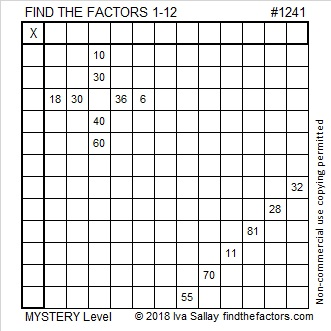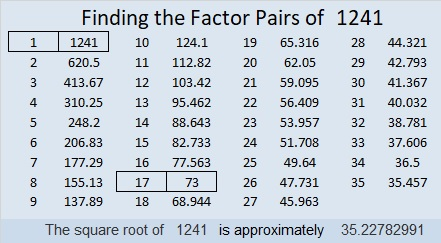# 1241 Mystery Level

Sure, you know many of the factors of the clues in this puzzle, but don’t write the first one that pops in your head. You might be lucky, but you also might have to do a lot of erasing if you do. Study all the clues and let logic tell you where to put each factor.Print the puzzles or type the solution in this excel file: 12 factors 1232-1241

Since this is the 1241st post, I’ll write a little about the number 1241:

• 1241 is a composite number.
• Prime factorization: 1241 = 17 × 73
• The exponents in the prime factorization are 1 and 1. Adding one to each and multiplying we get (1 + 1)(1 + 1) = 2 × 2 = 4. Therefore 1241 has exactly 4 factors.
• Factors of 1241: 1, 17, 73, 1241
• Factor pairs: 1241 = 1 × 1241 or 17 × 73
• 1241 has no square factors that allow its square root to be simplified. √1241 ≈ 35.227831241 is the sum of two squares two different ways:
29² + 20² = 1241
35² + 4² =1241

1241 is the hypotenuse of FOUR Pythagorean triples:
816-935-1241 which is 17 times (48-55-73)
584-1095-1241 which is (8-15-17) times 73
280-1209-1241 calculated from 2(35)(4), 35² – 4², 35² + 4²
441-1160-1241 calculated from 29² – 20², 2(29)(20), 29² + 20²